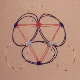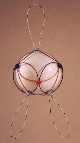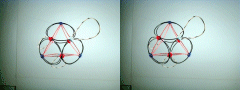# Neutron 3: 2nd Degree, Polar Star (N3-23P)This particular neutron shell is structurally identical to the second degree P3 shell, except that it has three P.V.B.'s pinned to it to increase the complement of Down quarks to six. This shell can easily be converted to a third degree N3 by removing all three of the radical strings (P.V.B's) and replacing them with a single polar circlet.Required Snap Points: 33 Available Snap Points: 30 Pinned Vector Bosons: 3 L1 = 19.36958654" (Structural) L2 = 15.81520673" (Filler) Model Diameter: 7.11934433" Loop Ratio (1:2): 1.224744442 Loop Equation: 3(L1) + 6(L2) = 153" Snap Point Equation: 3(4) + 6(3) = 30 A.S.P. PVB's:(+ 3 P.V.B. = 33 R.S.P.)

## Stereograms of N3-23P Shell# Neutron 3: 3rd Degree, Polar Star (N3-30P)

 This is the data referred to above for an N3 shell with no PVB's. The three radical strings are plucked off the model shown above and replaced with a single polar circlet, this puts three Down quarks at one pole and three Up quarks at the other pole. (No photo... solved on paper.)

 Required Snap Points: 33 Available Snap Points: 33 Pinned Vector Bosons: 0 L1 = 18.05026111" (Structural) L2 = 14.73798165" (Filler) L3 = 10.42132676" (Polar) Model Diameter: 6.634422673" Loop Ratios: (1:2): 1.224744442 (1:3): 1.732050201 (2:3): 1.414213563 Loop Equation: 3(L1) + 6(L2) + 1(L3) = 153" Snap Point Equation: 3(4) + 6(3) + 1(3) = 33 R.S.P.

Copyright 1997 by Arnold J. Barzydlo
Go Back